# The Calculator Encyclopedia Computes the Significant Figures of a Number

According to Wikipedia,

The significant figures (also known as the significant digits) of a number are digits that carry meaning contributing to its measurement resolution. This includes all digits except:

• All leading zeros;
• Trailing zeros when they are merely placeholders to indicate the scale of the number (exact rules are explained at identifying significant figures); and
• Spurious digits introduced, for example, by calculations carried out to greater precision than that of the original data, or measurements reported to a greater precision than the equipment supports.

Nickzom Calculator computes the significant figures of any given number.

For Example: apply 3 significant figures to 123876  and apply 2 significant figures to 0.00009872.

First, you need to obtain the Nickzom Calculator – The Calculator Encyclopedia app.
You can get this app via any of these means:

Once, you have obtained the calculator encyclopedia app, proceed to the Calculator Map, then click on Significant Figures under the Mathematics sectionOn clicking the page or activity to enter the values is displayed.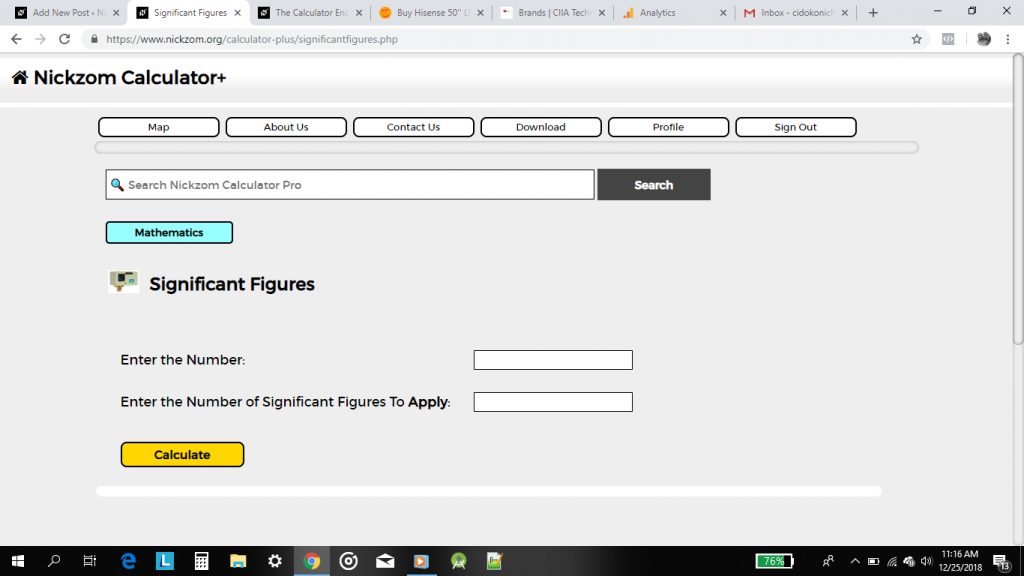From the first example: apply 3 significant figures to 123876
It implies that the number is 123876 and the number of significant figures to apply is 3.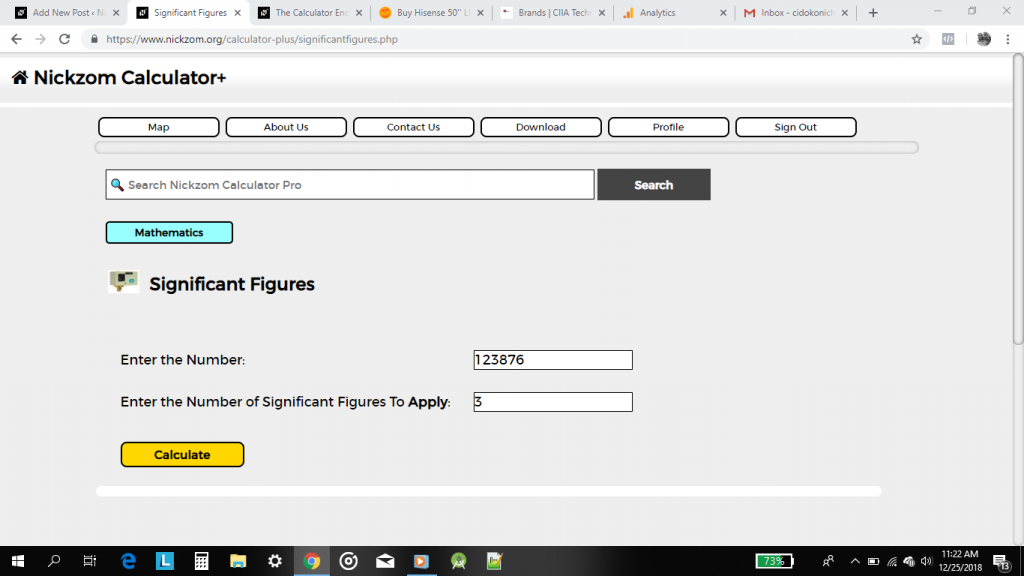Click on the Calculate button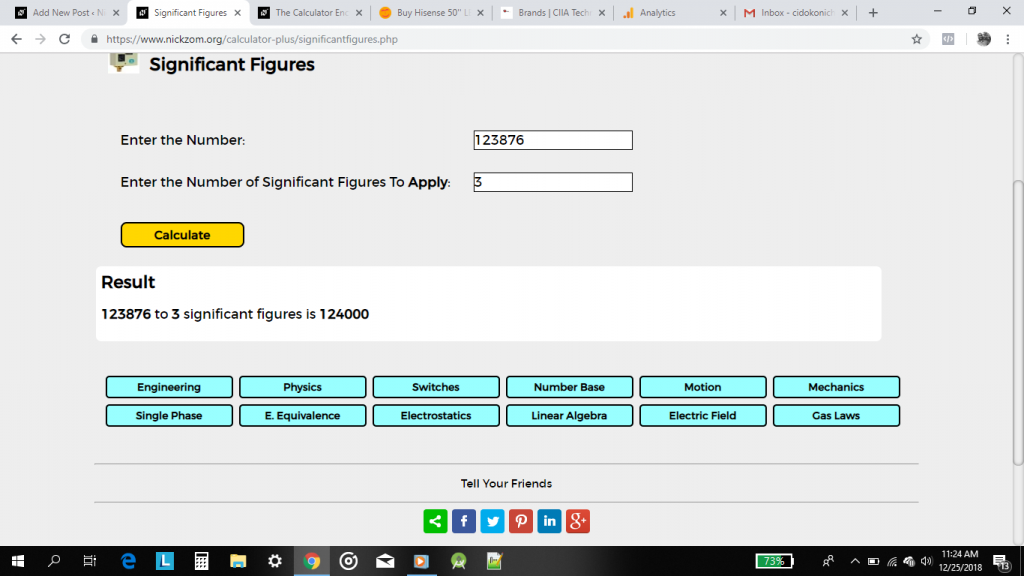Now, let’s run through the next example: apply 2 significant figures to 0.00009872.

It implies that the number is 0.00009872 and the number of significant figures to apply is 2.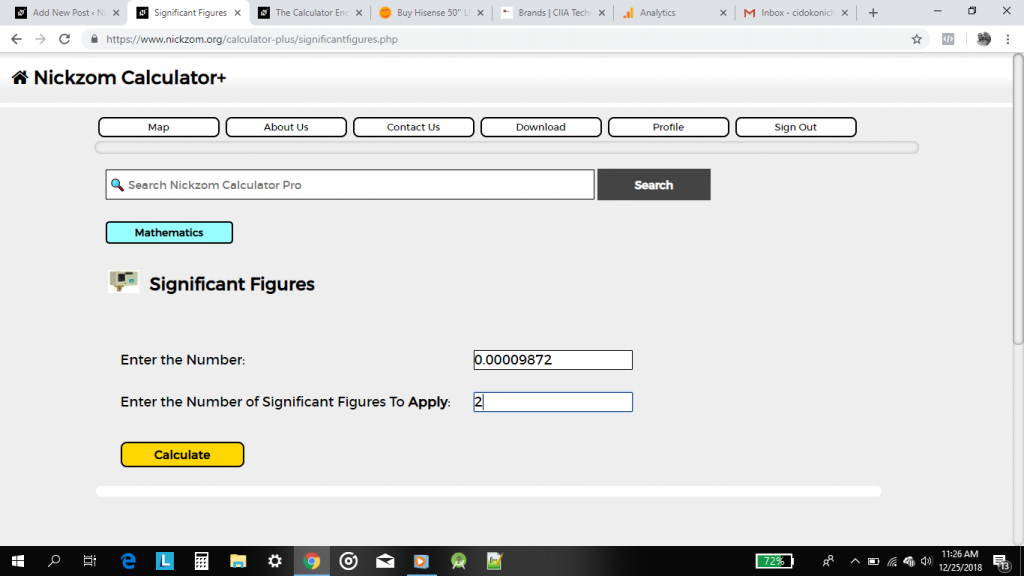Lastly, click on the Calculate button.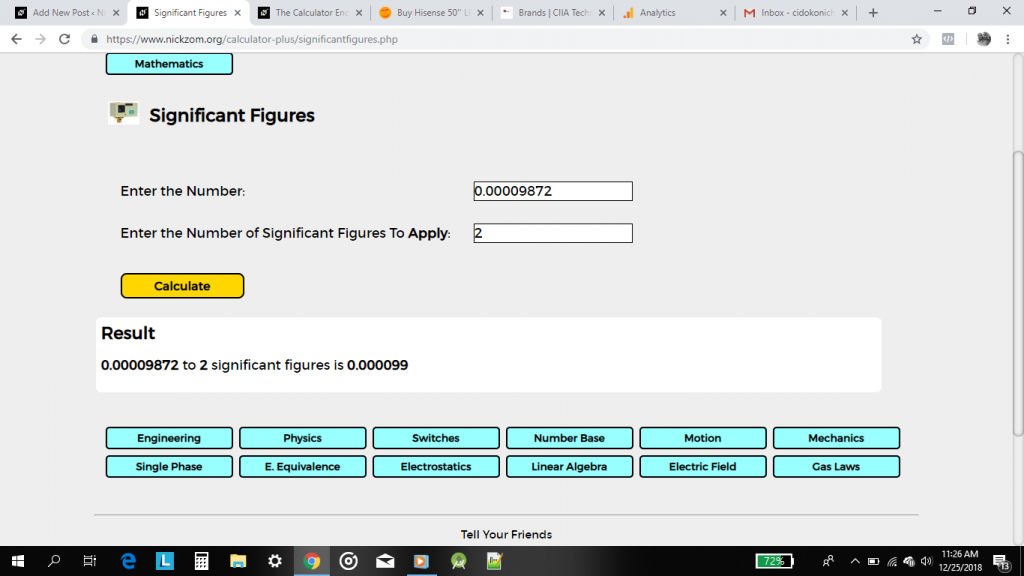Life is made easier and faster with The Calculator Encyclopedia – Nickzom Calculator.